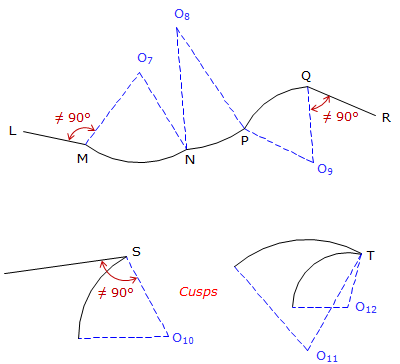## 3. Tangency Condition

At its ends, an arc can be joined to a straight line or another arc. At the connection point, the relationship with the adjacent boundary can be tangent or non tangent.

For a tangent condition, Figure F-5,

Line to arc (and arc to line) - line is perpendicular to the radius at the intersection.

Arc to Arc - intersection point and radius point of both arcs are collinear; all three point are on a straight line.Figure F-5 Tangent Conditions

Any deviation from these constraints results in a non-tanget transition, Figure F-6.Figure F-6 Nontangent Conditions